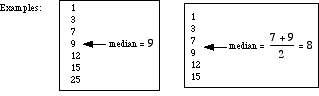# median of a set of numbers

Median of a Set of Numbers

The median of a set of numbers is the value for which half the numbers are larger and half are smaller. If there are two middle numbers, the median is the arithmetic mean of the two middle numbers.

Note: The median is a good choice to represent the center of a distribution when the distribution is skewed or has outliers.